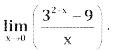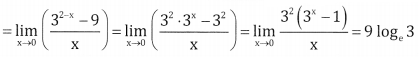# 2nd PUC Basic Maths Question Bank Chapter 17 Limit and Continuity of a Function Ex 17.3

Students can Download Basic Maths Exercise 17.3 Questions and Answers, Notes Pdf, 2nd PUC Basic Maths Question Bank with Answers helps you to revise the complete Karnataka State Board Syllabus and score more marks in your examinations.

## Karnataka 2nd PUC Basic Maths Question Bank Chapter 17 Limit and Continuity of a Function Ex 17.3

Part – A

2nd PUC Basic Maths Limit and Continuity of a Function Ex 17.3 One and Two Marks Questions and Answers

Question 1.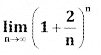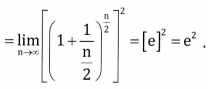Question 2.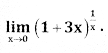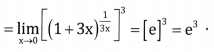Question 3.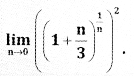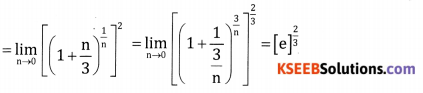Question 4.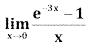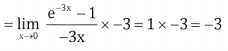Question 5.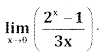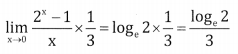Part – B

2nd PUC Basic Maths Limit and Continuity of a Function Ex 17.3 Three Marks Questions and Answers

Question 1.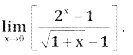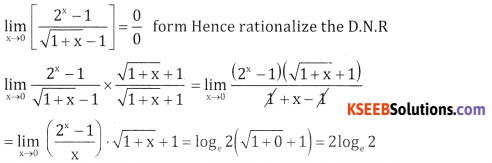Question 2.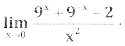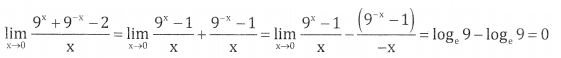Question 3.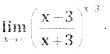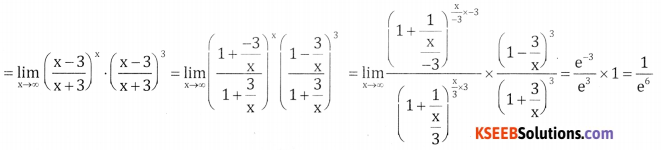Question 4.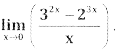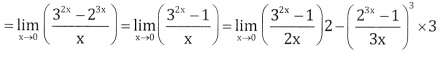= log 9 – log 8 = log $$\frac { 9 }{ 8 }$$